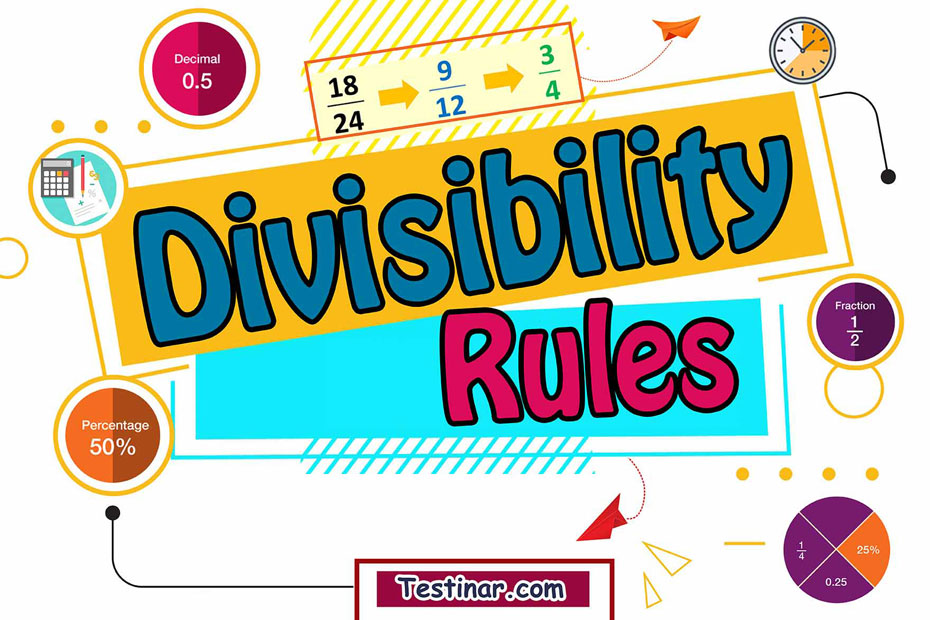## What are the Divisibility Rules

Any number is said to be completely divisible by another number if it leaves no remainder. For example, we can say that 12 is completely divisible by 2, but 12 is not completely divisible by 7. So, for these reasons, its very important to know the divisibility rules for all numbers ranging from 2-9. Moreover, these rules come in handy when dividing large numbers without going into the conventional division rules.

Also, these rules will help you determine whether a number is divisible by another number or not, in a jiffy!

#### Divisibility Rules

• Divisible by 1 Each and every number in the universe is divisible by 1.
• Divisible by 2 A number is only divisible by 2, if its last digit is even.
• Divisible by 3 If the sum of all digits of a number is divisible by 3, then the number itself is divisible by 3.
• Divisible by 4 A number is usually divisible by 4, if its last 2 digits are divisible by 4.
• Divisible by 5 To be divisible by 5, a number must contain the last digits as either 0 or 5.
• Divisible by 6 Any number which is divisible by both 2 and 3 will also be divisible by 6.
• Divisible by 7 To find if a number is divisible by 7, just check if by doubling the last digit of the number and then subtracting it from the remaining digit is divisible by 7 or not, the process for checking divisibility by 7 may need to be repeated multiple times for larger numbers, as the first operation may not yield a number that is obviously divisible by 7
• Divisible by 8 A number is divisible by 8, only if the last 3 digits of the number is divisible by 8.
• Divisible by 9 Any number whose sum of digits is divisible by 9, is itself divisible by 9.
• Divisible by 10 For a number to be divisible by 10, it should have the last digit (the digit at the one’s place) as zero.

For example, Let’s check if 49 is divisible by 7 or not.
So, last digit of 49 is 9 and double of 9 is 18. Now 18-4=14 which is divisible by 7. Hence, 49 is divisible by 7.

### Exercises for Divisibility Rules

1) Is $$8596742$$ divisible by $$2$$?

2) Is $$852$$ divisible by $$3$$?

3) Is $$8522$$ divisible by $$4$$?

4) Is $$852205$$ divisible by $$5$$?

5) Is $$348$$ divisible by $$7$$?

6) Is $$3241$$ divisible by $$9$$?

1) Is $$8596742$$ divisible by $$2$$?
Solution
Yes, because the last digit is an even number, so $$8596742$$ is divisible by $$2$$
2) Is $$852$$ divisible by $$3$$?
Solution
Yes, because the sum of its digits is  $$15$$ and $$15$$ is divisible by 3, therefore $$8596742$$ is divisible by $$3$$.
3) Is $$8522$$ divisible by $$4$$?

Solution
No, because the number represented by its last two digits that is $$22$$ is not divisible by $$4$$ , therefore $$8522$$ is not divisible by $$4$$
4) Is $$852205$$ divisible by $$5$$?
Solution
Yes, because its last digit is 5, therefore $$852205$$ is divisible by $$5$$.
5) Is $$348$$ divisible by $$7$$?

Solution
No, because if we double the last digit of the number $$8 \times 2 = 16$$ , and then subtract it from the remaining number: $$34 -16 =18$$ , the result is not divisible by 7, therefore $$348$$ is not divisible by $$7$$.
6) Is $$3241$$ divisible by $$9$$?
Solution
No, because if the sum of its digits is divisible by $$9$$. In this number the sum of digits is $$3+2+4+1 =10$$ and it is not divisible by $$10$$ , therefore $$3241$$ is not divisible by $$9$$.

## Divisibility Rules Quiz

### GED Math Full Study Guide 2022-2023

$25.99$13.99

### The Most Comprehensive ATI TEAS 6 Math Preparation Bundle

$76.99$36.99

### ALEKS Math Full Study Guide

$25.99$12.99

### HiSET Mathematics Formulas

$6.99$5.99